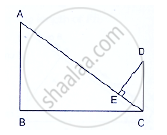Share

Books Shortlist
Your shortlist is empty

# Solution for In Figure Below, If Ab ⊥ Bc, Dc ⊥ Bc and De ⊥ Ac, Prove that δ Ced ~ Abc. - CBSE Class 10 - Mathematics

#### Question

In Figure below, if AB ⊥ BC, DC ⊥ BC and DE ⊥ AC, Prove that Δ CED ~ ABC.Given: AB ⊥ BC, DC ⊥ BC and DE ⊥ AC

To prove: ΔCED ~ ΔABC

Proof:

∠BAC + ∠BCA = 90°                  …(i) [By angle sum property]

And, ∠BCA + ∠ECD = 90°             …(ii) [DC ⊥ BC given]

Compare equation (i) and (ii)

∠BAC = ∠ECD                           …(iii)

In ΔCED and ΔABC

∠CED = ∠ABC                        [Each 90°]

∠ECD = ∠BAC                         [From (iii)]

Then, ΔCED ~ ΔABC               [By AA similarity]

This question doesn't have a solution currently. Please check again in a few days.
Is there an error in this question or solution?

#### Video TutorialsVIEW ALL 

Solution for question: In Figure Below, If Ab ⊥ Bc, Dc ⊥ Bc and De ⊥ Ac, Prove that δ Ced ~ Abc. concept: Triangles Examples and Solutions. For the course CBSE
S